##### The Japanese Bonsai specialist
Direct order Contact Help / Services Newsletter# Bamboo water dispenser for tsukubai bassin

› Bamboo fences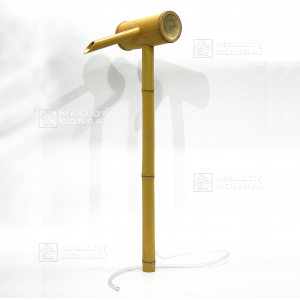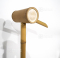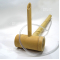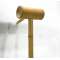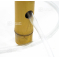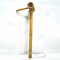ref. : 4559

36,00

voluminous/heavy item extra shipping of 6,00

This item is temporarily unavailableNotify availability

###### Description

Length / height: + - 800 Ø mm 40 mm. Length of the beak spout 210 Ø mm of the beak spout: + -20/25 mm. This article of traditional manufacture, is used over in decoration to the of the stone to water and basins of granite.Sold equipped of a hose plastic diameter + - 10 mm outside and 8 mm inside transporting water until his/her beak. Sold without pump. To see on this boutique different models: www.conrad.fr

#bamboo 4.6 #water 4.5 #beak 3.4 #dispenser 2.9 #tsukubai 2.8 #fences 2.6 #length 2.6 #bassin 2.6 #spout 2.5 #this 2.4

Formule
(( ROUND((CHAR_LENGTH(b.article_nom)-CHAR_LENGTH(REPLACE(b.article_nom, 'water', '')))/LENGTH('water')) + ROUND((CHAR_LENGTH(b.article_description)-CHAR_LENGTH(REPLACE(b.article_description, 'water', '')))/LENGTH('water')) ) * 4.5) + (( ROUND((CHAR_LENGTH(b.article_nom)-CHAR_LENGTH(REPLACE(b.article_nom, 'beak', '')))/LENGTH('beak')) + ROUND((CHAR_LENGTH(b.article_description)-CHAR_LENGTH(REPLACE(b.article_description, 'beak', '')))/LENGTH('beak')) ) * 3.4) + (( ROUND((CHAR_LENGTH(b.article_nom)-CHAR_LENGTH(REPLACE(b.article_nom, 'dispenser', '')))/LENGTH('dispenser')) + ROUND((CHAR_LENGTH(b.article_description)-CHAR_LENGTH(REPLACE(b.article_description, 'dispenser', '')))/LENGTH('dispenser')) ) * 2.9) + (( ROUND((CHAR_LENGTH(b.article_nom)-CHAR_LENGTH(REPLACE(b.article_nom, 'tsukubai', '')))/LENGTH('tsukubai')) + ROUND((CHAR_LENGTH(b.article_description)-CHAR_LENGTH(REPLACE(b.article_description, 'tsukubai', '')))/LENGTH('tsukubai')) ) * 2.8) + (( ROUND((CHAR_LENGTH(b.article_nom)-CHAR_LENGTH(REPLACE(b.article_nom, 'bassin', '')))/LENGTH('bassin')) + ROUND((CHAR_LENGTH(b.article_description)-CHAR_LENGTH(REPLACE(b.article_description, 'bassin', '')))/LENGTH('bassin')) ) * 2.6) + (( ROUND((CHAR_LENGTH(b.article_nom)-CHAR_LENGTH(REPLACE(b.article_nom, 'bamboo', '')))/LENGTH('bamboo')) + ROUND((CHAR_LENGTH(b.article_description)-CHAR_LENGTH(REPLACE(b.article_description, 'bamboo', '')))/LENGTH('bamboo')) ) * 2.6) + (( ROUND((CHAR_LENGTH(b.article_nom)-CHAR_LENGTH(REPLACE(b.article_nom, 'length', '')))/LENGTH('length')) + ROUND((CHAR_LENGTH(b.article_description)-CHAR_LENGTH(REPLACE(b.article_description, 'length', '')))/LENGTH('length')) ) * 2.6) + (( ROUND((CHAR_LENGTH(b.article_nom)-CHAR_LENGTH(REPLACE(b.article_nom, 'spout', '')))/LENGTH('spout')) + ROUND((CHAR_LENGTH(b.article_description)-CHAR_LENGTH(REPLACE(b.article_description, 'spout', '')))/LENGTH('spout')) ) * 2.5) + (( ROUND((CHAR_LENGTH(b.article_nom)-CHAR_LENGTH(REPLACE(b.article_nom, 'sold', '')))/LENGTH('sold')) + ROUND((CHAR_LENGTH(b.article_description)-CHAR_LENGTH(REPLACE(b.article_description, 'sold', '')))/LENGTH('sold')) ) * 2.4) + (( ROUND((CHAR_LENGTH(b.article_nom)-CHAR_LENGTH(REPLACE(b.article_nom, 'this', '')))/LENGTH('this')) + ROUND((CHAR_LENGTH(b.article_description)-CHAR_LENGTH(REPLACE(b.article_description, 'this', '')))/LENGTH('this')) ) * 2.4)

## Secure payment## Delivery

Our logistic partners :04 74 55 23 48
Pépinière MAILLOT-BONSAÏ
Le Bois Frazy
01990 RELEVANT - FRANCE
on appointment